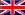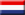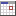# Online calculation of the interest payment as banks do.

Select language:This tool calculates the interest rate as most banks do. This service uses the "daily interest calculation" method where the actual number of days in the month is used and one year is set at 365 days and in a leap year 366 days.

To calculate the interest rate the following input data are required:
• A list of amounts to and from a savings account with matching deposit or withdrawal dates.
The dates must be value dates and no posting dates.
• A list of interim interest changes with corresponding dates.
This is especially the case if you have a savings account with a variable interest rate.
• A list of dates when interest is credited to the savings account.
Example savings account with deposits and withdrawals and interest rate changes:
1. On 24-03-2007 your balance in your savings account is 10000.00 and the interest rate is 4.50%.
• balance = 10000.00
• interest days = 35
• interest numbers = 10000.00 / 100 * 35 = 3500
• interest amount = 3500 * 4.50 / 365 = 43.1507
• total accrued interest = 43.1507
2. On 28-04-2007 1000.00 is deposited.
• balance = 10000.00 + 1000.00 = 11000.00
• interest days = 58
• interest numbers = 11000.00 / 100 * 58 = 6380
• interest amount = 6380 * 4.50 / 365 = 78.6575
• total accrued interest = 43.1507 + 78.6575 = 121.8082
3. On 25-06-2007 the interest rate is changed to 4.00%.
• balance = 11000.00
• interest days = 82
• interest numbers = 11000.00 / 100 * 82 = 9020
• interest amount = 9020 * 4.00 / 365 = 98.8493
• total accrued interest = 121.8082 + 98.8493 = 220.6575
4. On 15-09-2007 2000.00 is withdrawn.
• balance = 11000.00 - 2000.00 = 9000.00
• interest days = 76
• interest numbers = 9000.00 / 100 * 76 = 6840
• interest amount = 6840 * 4.00 / 365 = 74.9589
• total accrued interest = 220.6575 + 74.9589 = 295.6164
5. On 30-11-2007 interest is credited to your savings account.
• interest paid on savings account = 295.6164
• balance = 9000.00 + 295.6164 = 9295.62
• interest days = 31
• interest numbers = 9295.62 / 100 * 31 = 2881.6422
• interest amount = 2881.6422 * 4.00 / 365 = 31.5796
• total accrued interest = 31.5796
6. On 31-12-2007 the interest rate remains unchanged at 4.00%.
Note: When transitioning to another year, always specify the interest rate on Dec 31.
• balance = 9295.62
• interest days = 1
• interest numbers = 9295.62 / 100 * 1 = 92.9562
• interest amount = 92.9562 * 4.00 / 365 = 1.0187
• total accrued interest = 31.5796 + 1.0187 = 32.5983
7. On 1-1-2008 the interest rate remains unchanged at 4.00%.
Note: When transitioning from another year, always specify the interest rate on Jan 1.
• balance = 9295.62
• interest days = 9
• interest numbers = 9295.62 / 100 * 9 = 836.6058
• interest amount = 836.6058 * 4.00 / 366 (leap year) = 9.1432
• total accrued interest = 32.5983 + 9.1432 = 41.7415
8. On 10-1-2008 3000.00 is deposited.
• balance = 9295.62 + 3000.00 = 12295.62
• interest days = 112
• interest numbers = 12295.62 / 100 * 112 = 13771.0944
• interest amount = 13771.0944 * 4.00 / 366 = 150.5038
• total accrued interest = 41.7415 + 150.5038 = 192.2453
9. On 1-05-2008 the interest rate is changed to 3.50%.
• balance = 12295.62
• interest days = 108
• interest numbers = 12295.62 / 100 * 108 = 13279.2696
• interest amount = 13279.2696 * 3.50 / 366 (leap year) = 126.9876
• total accrued interest = 192.2453 + 126.9876 = 319.2329
10. On 17-8-2008 5000.00 is deposited.
• balance = 12295.62 + 5000.00 = 17295.62
• interest days = 105
• interest numbers = 17295.62 / 100 * 105 = 18160.4010
• interest amount = 18160.4010 * 3.50 / 366 (leap year) = 173.6650
• total accrued interest = 319.2329 + 173.6650 = 492.8979
11. On 30-11-2008 interest is credited to your savings account.
• interest paid on savings account = 492.8979
• balance = 17295.62 + 492.8979= 17788.52
• interest days = 31
• interest numbers = 17788.52 / 100 * 31 = 5514.4412
• interest amount = 5514.4412 * 3.50 / 366 (leap year) = 52.7337
• total accrued interest = 52.7337
12. The final date of the calculation is 31-12-2008.
• balance = 17788.52
• total accrued interest = 52.7337
Explanation balance:
The balance are all deposits and withdrawals added together at the relevant date. If on the same date interest is credited to the savings account, the interest is added to the balance.

Explanation interest days:
In calculating the number of days (interest days) the first day shall be counted but not the last day. In the example above, March 24 (step 1) is counted but the next date April 28 (step 2) is not. The number of days (interest days) during that period is 35.

Explanation interest numbers:
Banks use interest numbers when interim interest rate changes take place and there are many deposits and withdrawals. The interest numbers are calculated by dividing the balance by 100 and then multiplied by the number of interest days.

Explanation interest amount:
The interest amount is calculated by multiplying the interest number with the interest rate and then dividing it by 365 (in a leap year by 366).
Note: The specified date is used to determine whether it is a leap year or not.

Explanation total accrued interest and interest paid on savings account:
The total accrued interest are all interest amounts added together at the relevant date.
The interest that is credited to the savings account is the total accrued interest from the previous period (see step 5 and 10). Once the interest is credited to the savings account, the total accrued interest is set to 0 plus current calculated interest amount.

Explanation final date:
The interest calculation starts from the first transaction date in field 1 until and including the end date you specify separately. If you had put the end date on 30-11-2007 in the example above, the interest calculation would start from 24-03-2007 until and including 30-11-2007.

YOU USE THIS SERVICE AT YOUR OWN RISK. MOBILEFISH.COM WILL NOT BE LIABLE FOR ANY DATA LOSS, DAMAGES, LOSS OF PROFITS OR ANY OTHER KIND OF LOSS WHILE USING OR MISUSING THIS SERVICE.

## Input calculation of the interest payment:

 Field 1Savings deposits / withdrawals (Max. 1000 )*:Field 2Savings interest rates changes (Max. 1000 )*:Field 3Payment dates interest savings account (Max. 366 )*:Search and replace:Search replace with in Field 1 Field 2 Date format *:dd-mm-yyyy or dd/mm/yyyymm-dd-yyyy or mm/dd/yyyy in Field 1, 2 and 3 Delimiter used*:;[tab]|~=^_ in Field 1 and 2 Final date*:To prevent automated submissions an Access Code has been implemented for this tool.Please enter the Access Code as displayed above. *: * = required